# Aptitude - Height & Distance Online Quiz

Following quiz provides Multiple Choice Questions (MCQs) related to Height & Distance. You will have to read all the given answers and click over the correct answer. If you are not sure about the answer then you can check the answer using Show Answer button. You can use Next Quiz button to check new set of questions in the quiz.Q 1 - A man on the top of a vertical observation tower observes a car moving at a uniform speed coming directly towards it. If it takes 10 minutes for the angle of depression to change from 45° to 60°, how soon after this will the car reach the observation tower?

A - 10 min 30 sec

B - 13 min 20 sec

C - 15 min 10 sec

D - 16 min 30 sec

### Explanation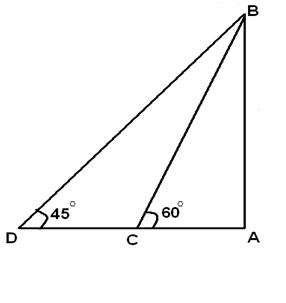```Let AB be the tower and C and D be the two positions of the car.
Then,from figure
AB/AC=tan 60 =√3 => AB=√3AC
AB=AC+CD
CD=AB-AC=√3AC - AC=AC (√3-1)
CD = AC (√3-1) =>10 min
AC=> ?
AC/(AC(√3-1)) x 10=?=10/(√3-1)=13.66=13 min 20 sec(approx)```

Q 2 - An aeroplane when 750 m high passes vertically above another aeroplane at an instant when their angles of elevation at same observing point are 45° and 30&de; respectively. Approximately, how many meters higher is the one than the other?

A - 250(1- √3)

B - 750(3- √3)

C - 250(3- √3)

D - 275(1- √3)

### Explanation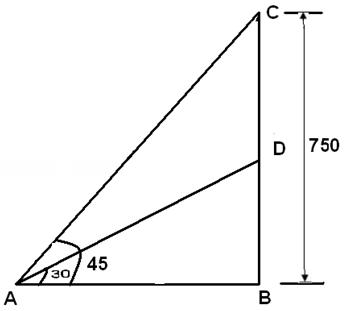```Let C and D be the position of the aeroplanes.
Given that CB = 900 m,∠CAB = 60°,∠DAB = 45°
From the right △ ABC,
Tan45=CB/AB=>CB=AB
Tan30=DB/AB=>DB=ABtan30=CBx(1/√3)=750/√3
CB=CD+DB
=> Required height CD=CB-DB=750-750/√3=250(3- √3)
```

Q 3 - A straight tree is broken due to thunder storm. The broken part is bent in such a way that the peak touches the ground at an angle elevation of 45°. The distance of peak of tree (where it touches the root of the tree is 20 m. Then the height of the tree is

A - 48.28 meters

B - 30.28 meters

C - 24.14 meters

D - 28.14meters

### Explanation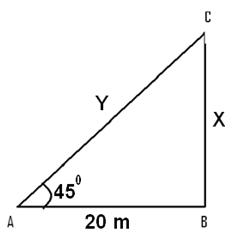```Let the total length of the tree be X+Y meters
From the figure tan 45=X/20 =>X=20
cos 45 = 20/Y =>Y=20/cos 45 =20√2

Q 4 - The shadow of a building is 10 m long when the point of rise of the sun is 60°. Discover the building's stature.

A - 16.32m

B - 17.32 m

C - 18.32 m

D - 19.32m

### Explanation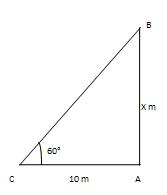```Let AB be the building and AC be its shadow.
Then, AC=20m and ∠ACB=60°.Let AB= x m.
Presently AB/AC=tan 60°=√3=>x/10=√3
=>x=10√3m= (10*1.732) m=17.32m.
∴ Height of the building is 17.32m.```

Q 5 - From The highest point of a 10 m high building, the edge of rise of the of the highest point of a tower is 60° and the despondency's edge of its foot is 45°,Find The tower's stature. (take√3=1.732)

A - 24.3m

B - 25.3m

C - 26.3 m

D - 27.3 m

### Explanation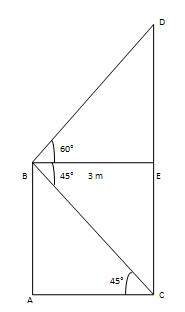```Let AB be the building and CD be the tower.
Draw BE perpendicular to CD.
At that point CE =AB = 10m, ∠EBD= 60° and ∠ACB= ∠ CBE=45°
AC/AB= cot45°=1 = >AC/10 =1 => AC = 10m.
From △ EBD, we have
DE/BE= tan 60°=√3 => DE/AC= √3
=> DE/10= 1.732 =>DE = 17.3
Height of the tower = CD= CE+DE= (10+17.32) = 27.3 m.```

Q 6 - At a moment, the shadow's length of a shaft is √3 times the stature of the shaft. The edge of rise of the sun is:

A - 30°

B - 45°

C - 60°

D - 75°

### Explanation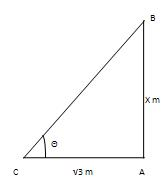```Let AB be the post and AC be its shadow.
Let AB =X m. Then, AC= √3Xm.Let∠ACB=θ.
AB/AC=tanθ => tanθ = X/√3X = 1/√3 =tan30°.
∴ θ = 30°.
Hence, the point of rise is 30°.```

Q 7 - A kite is flying at a tallness of 75 m from the level of ground, joined to a string slanted at 60° to the level. The string's length is:

A - 50 √2 m

B - 50√3 m

C - 50m/√2

D - 50m/√3

### Explanation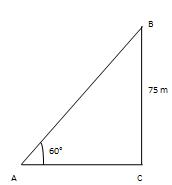```Let AB be the kite and AC be the level ground
So that BC - AC.
At that point, ∠BAC=60°and BC=75m. Let AB=x meters.
Presently AB/BC=coses60°=2/ √3
=> x/75=2/√3 =>x=150/√3 =150* √3/3=50 √3m.
∴ Length of the string=50 √3m.```

Q 8 - On the level plane, there is a vertical tower with a flagpole on its top. At a point 9m far from the tower, the edges of rise of the top and Base of the flagpole are 60°and 30°respectively.The flagpole's tallness is:

A - 6 √3 m

B - 5 √3m

C - 6 √2m

D - 4m

### Explanation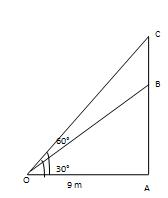```Let AB be the tower and BC be the flag pole and let O be the point of observation.
Then, A=9m, ∠AOB=30°and ∠AOC=60°
AB/OA=tan30°=1 ∠ =>AB/9=1∠
=>AB=(9*1/ √3* √3/√3)= 3 √3m.
AC/AO=tan60°=√3 =>AC/9= √3 =>AC= 9√3m.
∴BC= (AC-AB) = (9 √3-3 √3) m=6 √3m.
∴ Height of the flagpole is 6 √m.```

aptitude_height_distance.htm